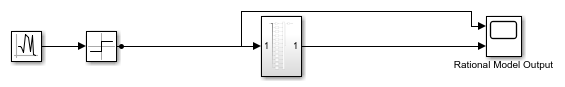Documentation

This example shows how to use Simulink® to simulate a differential high-speed backplane channel. The example first reads a Touchstone® data file that contains single-ended 4-port S-parameters for a differential high-speed backplane and converts them to 2-port differential S-parameters. It computes the transfer function of the differential circuit and uses the `rationalfit` function to fit a closed-form rational function to the circuit's transfer function. Then, the example converts the poles and residues of the rational function object into the numerators and denominators of the Laplace Transform S-Domain transfer functions that it uses to build the Simulink model of the rational function object.

To run this example, you must have Simulink installed.

### Read the Single-Ended 4-Port S-Parameters and Convert Them to Differential 2-Port S-Parameters

Read a Touchstone data file, `default.s4p`, into an `sparameters` object. The parameters in this data file are the 50-ohm S-parameters of a single-ended 4-port passive circuit, measured at 1496 frequencies ranging from 50 MHz to 15 GHz. Then, get the single-ended 4-port S-parameters from the data object, and use the matrix conversion function `s2sdd` to convert them to differential 2-port S-parameters.

```filename = 'default.s4p'; backplane = sparameters(filename); data = backplane.Parameters; freq = backplane.Frequencies; z0 = backplane.Impedance; ```

Convert to 2-port differential S-parameters. This operation pairs together odd-numbered ports first, followed by the even-numbered ports. If a different configuration has been used to measure the single ended S-parameters, you can specify a different second argument in the s2sdd command. For example, option "2" will allow you to pair the input and output ports in ascending order. Alternatively, you can use the command snp2smp to change the port order.

```diffdata = s2sdd(data,1); diffz0 = 2*z0; ```

### Compute the Transfer Function and Its Rational Function Representation

First, use the `s2tf` function to compute the differential transfer function. Then, use the `rationalfit` function to compute the closed form of the transfer function and store it in an `rfmodel.rational` object. The `rationalfit` function fits a rational function object to the specified data over the specified frequencies.

```difftf = s2tf(diffdata,diffz0,diffz0,diffz0); fittol = -30; % Rational fitting tolerance in dB delayfactor = 0.9; % Delay factor rationalfunc = rationalfit(freq,difftf,fittol,'DelayFactor', delayfactor) npoles = length(rationalfunc.A); fprintf('The derived rational function contains %d poles.\n', npoles); ```
```rationalfunc = rfmodel.rational with properties: A: [20x1 double] C: [20x1 double] D: 0 Delay: 6.0172e-09 Name: 'Rational Function' The derived rational function contains 20 poles. ```

### Get the Numerator and Denominator of the Laplace Transform S-Domain Transfer Functions

This example uses Laplace Transform S-Domain transfer functions to represent the backplane in the Simulink model. Convert the poles and corresponding residues of the rational function object into numerator and denominator form for use in the Laplace Transform transfer function blocks. Each transfer function block represents either one real pole and the corresponding real residue, or a pair of complex conjugate poles and residues, so the transfer function block always has real coefficients. For this example, the rational function object contains 2 real poles/residues and 6 pairs of complex poles/residues, so the Simulink model contains 8 transfer function blocks.

```A = rationalfunc.A; C = rationalfunc.C; den = cell(size(A)); num = cell(size(A)); k = 1; % Index of poles and residues n = 0; % Index of numerators and denominators while k <= npoles if isreal(A(k)) % Real poles n = n + 1; num{n} = C(k); den{n} = [1, -A(k)]; k = k + 1; else % Complex poles n = n + 1; real_a = real(A(k)); imag_a = imag(A(k)); real_c = real(C(k)); imag_c = imag(C(k)); num{n} = [2*real_c, -2*(real_a*real_c+imag_a*imag_c)]; den{n} = [1, -2*real_a, real_a^2+imag_a^2]; k = k + 2; end end den = den(1:n); num = num(1:n); ```

### Build the Simulink Model of the Backplane

Build a Simulink model of the backplane using the Laplace Transform transfer functions. Then, connect a random source to the input of the backplane and a scope to its input and output.

```modelname = fliplr(strtok(fliplr(tempname), filesep)); simulink_rfmodel_build_rational_system_helper(modelname , numel(num)) simulink_rfmodel_add_source_sink_helper(modelname) ```Figure 1. Simulink model for a rational function

### Simulate the Simulink Model of the Rational Function

When you simulate the model, the Scope shows the impact of the differential backplane on the random input signal.

```set_param([modelname,'/Rational Model Output'], 'Open', 'on') h = findall(0, 'Type', 'Figure', 'Name', 'Rational Model Output'); h.Position = [200, 216, 901, 442]; sim(modelname); ```### Close the Model

```close_system(modelname, 0) ```